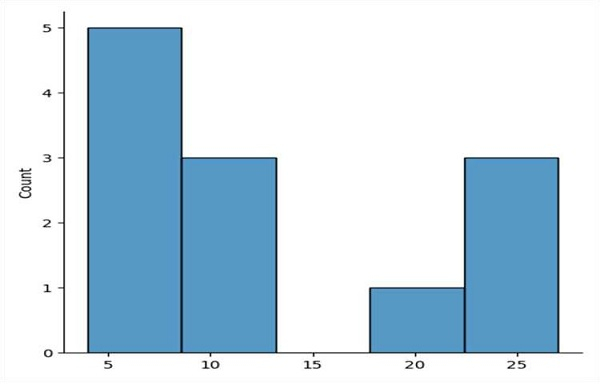# Plotting with seaborn using the matplotlib object-oriented interface

Seaborn is used to visualizing the random distribution and we can use matplotlib interface to show this distribution over a diagram.

We can take the following steps to show the diagram −

• Figure level interface for drawing distribution plots onto a Face Grid. This function provides access to several approaches for visualizing the univariate or bivariate distribution of data, including subsets of data defined by semantic mapping and faceting across multiple subplots.

• List of numbers can be passed in the above-defined method, i.e., displot().

• To show the diagram, plt.show() can be used whereas plot was drawn using Seaborn.

## Example

import matplotlib.pyplot as plt
import seaborn as sns

sns.displot([4, 5, 6, 7, 8, 9, 10, 11, 21, 23, 25, 27])

plt.show()

## Output